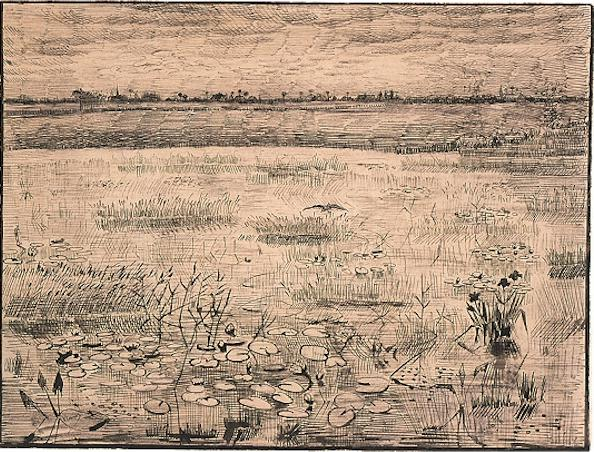# Luke 3:21-3821 One day when the crowds were being baptized, Jesus himself was baptized. As he was praying, the heavens opened, 22 and the Holy Spirit, in bodily form, descended on him like a dove. And a voice from heaven said, “You are my dearly loved Son, and you bring me great joy.”

23 Jesus was about thirty years old when he began his public ministry.

Jesus was known as the son of Joseph.
Joseph was the son of Heli.

24 Heli was the son of Matthat.
Matthat was the son of Levi.
Levi was the son of Melki.
Melki was the son of Jannai.
Jannai was the son of Joseph.

25 Joseph was the son of Mattathias. Mattathias was the son of Amos. Amos was the son of Nahum. Nahum was the son of Esli. Esli was the son of Naggai.

26 Naggai was the son of Maath. Maath was the son of Mattathias. Mattathias was the son of Semein. Semein was the son of Josech. Josech was the son of Joda 27 Joda was the son of Joanan. Joanan was the son of Rhesa. Rhesa was the son of Zerubbabel. Zerubbabel was the son of Shealtiel. Shealtiel was the son of Neri. 28 Neri was the son of Melki. Melki was the son of Addi. Addi was the son of Cosam. Cosam was the son of Elmadam. Elmadam was the son of Er. 29 Er was the son of Joshua. Joshua was the son of Eliezer. Eliezer was the son of Jorim. Jorim was the son of Matthat. Matthat was the son of Levi.

30 Levi was the son of Simeon. Simeon was the son of Judah. Judah was the son of Joseph. Joseph was the son of Jonam. Jonam was the son of Eliakim. 31 Eliakim was the son of Melea. Melea was the son of Menna. Menna was the son of Mattatha. Mattatha was the son of Nathan. Nathan was the son of David. 32 David was the son of Jesse. Jesse was the son of Obed. Obed was the son of Boaz. Boaz was the son of Salmon. Salmon was the son of Nahshon. 33 Nahshon was the son of Amminadab. Amminadab was the son of Admin. Admin was the son of Arni. Arni was the son of Hezron. Hezron was the son of Perez. Perez was the son of Judah.

34 Judah was the son of Jacob. Jacob was the son of Isaac. Isaac was the son of Abraham. Abraham was the son of Terah. Terah was the son of Nahor. 35 Nahor was the son of Serug. Serug was the son of Reu. Reu was the son of Peleg. Peleg was the son of Eber. Eber was the son of Shelah. 36 Shelah was the son of Cainan. Cainan was the son of Arphaxad. Arphaxad was the son of Shem. Shem was the son of Noah. Noah was the son of Lamech. 37 Lamech was the son of Methuselah. Methuselah was the son of Enoch. Enoch was the son of Jared. Jared was the son of Mahalalel. Mahalalel was the son of Kenan. 38 Kenan was the son of Enosh. Enosh was the son of Seth. Seth was the son of Adam. Adam was the son of God.